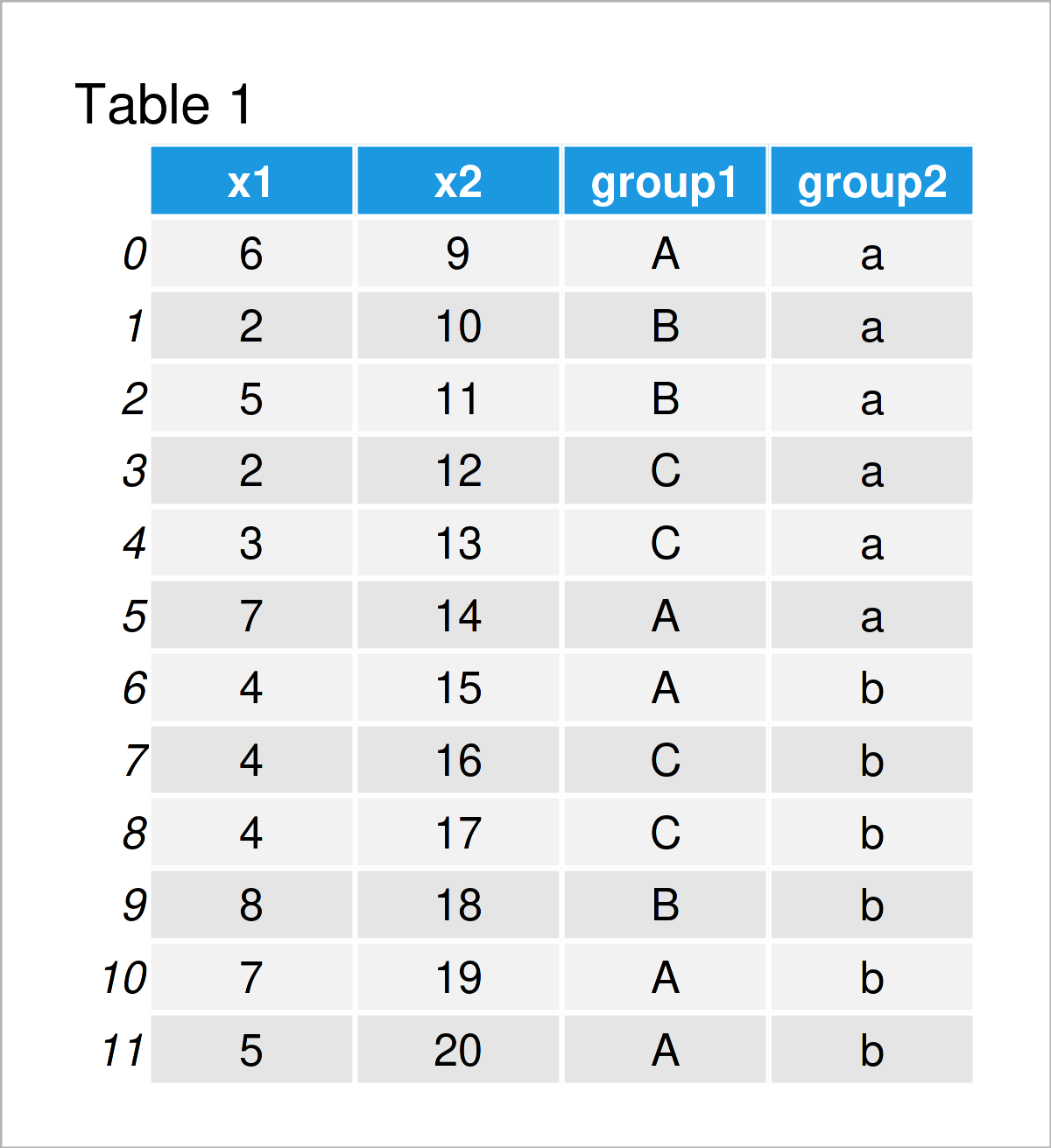# Quantile by Group in Python (2 Examples)

In this Python article you’ll learn how to get quantiles by group.

With that, let’s start right away…

## Example Data & Software Libraries

First, we have to import the pandas library:

`import pandas as pd                                              # Load pandas library`

We use the following pandas DataFrame as a basis for this Python programming tutorial:

```data = pd.DataFrame({'x1':[6, 2, 5, 2, 3, 7, 4, 4, 4, 8, 7, 5],  # Create pandas DataFrame
'x2':range(9, 21),
'group1':['A', 'B', 'B', 'C', 'C', 'A', 'A', 'C', 'C', 'B', 'A', 'A'],
'group2':['a', 'a', 'a', 'a', 'a', 'a', 'b', 'b', 'b', 'b', 'b', 'b']})
print(data)                                                      # Print pandas DataFrame```Have a look at the previous table. It shows that our exemplifying data is constituted of twelve rows and four variables.

## Example 1: Quantiles by Group in pandas DataFrame

In this example, I’ll demonstrate how to compute quantile values by group in a pandas DataFrame.

For this task, we can use the groupby and quantile functions as shown below:

```print(data.groupby('group1').quantile(0.25))              # Get first quartile by group
#           x1     x2
# group1
# A       5.00  14.00
# B       3.50  10.50
# C       2.75  12.75```

The previous output shows the first quartile of each group in each column.

Note that we could also calculate other types of quantiles such as deciles, percentiles, and so on. You can find more on this topic here.

## Example 2: Quantiles by Group & Subgroup in pandas DataFrame

This section illustrates how to find quantiles by two group indicators, i.e. a main and a subgroup.

For this task, we have to specify a list of group indicators within the groupby function:

```print(data.groupby(['group1', 'group2']).quantile(0.25))  # Get first quartile by multiple groups
#                  x1     x2
# group1 group2
# A      a       6.25  10.25
#        b       4.50  17.00
# B      a       2.75  10.25
#        b       8.00  18.00
# C      a       2.25  12.25
#        b       4.00  16.25```

## Video & Further Resources

Have a look at the following video on my YouTube channel. I demonstrate the content of this article in the video.

Furthermore, you might want to have a look at the related tutorials that I have published on my website. Some tutorials are shown below.

Summary: This tutorial has shown how to calculate quantiles by group in a pandas DataFrame in the Python programming language. In case you have additional questions, please let me know in the comments section below.

Subscribe to the Statistics Globe Newsletter